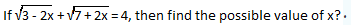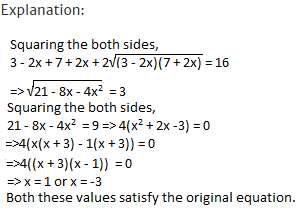UPSC  >  Test: Quadratic Equations- 2

# Test: Quadratic Equations- 2 - UPSC

Test Description

## 10 Questions MCQ Test CSAT Preparation - Test: Quadratic Equations- 2

Test: Quadratic Equations- 2 for UPSC 2023 is part of CSAT Preparation preparation. The Test: Quadratic Equations- 2 questions and answers have been prepared according to the UPSC exam syllabus.The Test: Quadratic Equations- 2 MCQs are made for UPSC 2023 Exam. Find important definitions, questions, notes, meanings, examples, exercises, MCQs and online tests for Test: Quadratic Equations- 2 below.
Solutions of Test: Quadratic Equations- 2 questions in English are available as part of our CSAT Preparation for UPSC & Test: Quadratic Equations- 2 solutions in Hindi for CSAT Preparation course. Download more important topics, notes, lectures and mock test series for UPSC Exam by signing up for free. Attempt Test: Quadratic Equations- 2 | 10 questions in 10 minutes | Mock test for UPSC preparation | Free important questions MCQ to study CSAT Preparation for UPSC Exam | Download free PDF with solutions
 1 Crore+ students have signed up on EduRev. Have you?
Test: Quadratic Equations- 2 - Question 1

### Find the value of a/b + b/a, if a and b are the roots of the quadratic equation x2 + 8x + 4 = 0?

Detailed Solution for Test: Quadratic Equations- 2 - Question 1

Explanation:

a/b + b/a = (a2 + b2)/ab = (a2 + b2 + a + b)/ab
= [(a + b)2 - 2ab]/ab
a + b = -8/1 = -8
ab = 4/1 = 4
Hence a/b + b/a = [(-8)2 - 2(4)]/4 = 56/4 = 14.

Test: Quadratic Equations- 2 - Question 2

###Detailed Solution for Test: Quadratic Equations- 2 - Question 2Test: Quadratic Equations- 2 - Question 3

### Find the quadratic equations whose roots are the reciprocals of the roots of 2x2 + 5x + 3 = 0?

Detailed Solution for Test: Quadratic Equations- 2 - Question 3

Explanation:

The quadratic equation whose roots are reciprocal of 2x2 + 5x + 3 = 0 can be obtained by replacing x by 1/x.
Hence, 2(1/x)2 + 5(1/x) + 3 = 0
=> 3x2 + 5x + 2 = 0

Test: Quadratic Equations- 2 - Question 4

A man could buy a certain number of notebooks for Rs.300. If each notebook cost is Rs.5 more, he could have bought 10 notebooks less for the same amount. Find the price of each notebook?

Detailed Solution for Test: Quadratic Equations- 2 - Question 4

Explanation:

Let the price of each note book be Rs.x.
Let the number of note books which can be brought for Rs.300 each at a price of Rs.x be y.
Hence xy = 300
=> y = 300/x
(x + 5)(y - 10) = 300 => xy + 5y - 10x - 50 = xy
=>5(300/x) - 10x - 50 = 0 => -150 + x2 + 5x = 0
multiplying both sides by -1/10x
=> x2 + 15x - 10x - 150 = 0
=> x(x + 15) - 10(x + 15) = 0
=> x = 10 or -15
As x>0, x = 10.

Test: Quadratic Equations- 2 - Question 5

I. a2 - 7a + 12 = 0,
II. b2 - 3b + 2 = 0 to solve both the equations to find the values of a and b?

Detailed Solution for Test: Quadratic Equations- 2 - Question 5

Explanation:

I.(a - 3)(a - 4) = 0
=> a = 3, 4

II. (b - 2)(b - 1) = 0
=> b = 1, 2
=> a > b

Test: Quadratic Equations- 2 - Question 6

I. a2 - 9a + 20 = 0,
II. 2b2 - 5b - 12 = 0 to solve both the equations to find the values of a and b?

Detailed Solution for Test: Quadratic Equations- 2 - Question 6

Explanation:

I. (a - 5)(a - 4) = 0
=> a = 5, 4
II. (2b + 3)(b - 4) = 0
=> b = 4, -3/2 => a ≥ b

Test: Quadratic Equations- 2 - Question 7

I. a2 + 11a + 30 = 0,
II. b2 + 6b + 5 = 0 to solve both the equations to find the values of a and b?

Detailed Solution for Test: Quadratic Equations- 2 - Question 7

Explanation:

I. (a + 6)(a + 5) = 0
=> a = -6, -5
II. (b + 5)(b + 1) = 0
=> b = -5, -1 => a ≤ b

Test: Quadratic Equations- 2 - Question 8

I. a2 + 8a + 16 = 0,
II. b2 - 4b + 3 = 0 to solve both the equations to find the values of a and b?

Detailed Solution for Test: Quadratic Equations- 2 - Question 8

Explanation:

I. (a + 4)2 = 0 => a = -4
II.(b - 3)(b - 1) = 0
=> b = 1, 3 => a < b

Test: Quadratic Equations- 2 - Question 9

I. a2 - 2a - 8 = 0,
II. b2 = 9 to solve both the equations to find the values of a and b?

Detailed Solution for Test: Quadratic Equations- 2 - Question 9

Explanation:

I. (a - 4)(a + 2) = 0
=> a = 4, -2
II. b2 = 9
=> b = ± 3
-2 < 3, -2 > -3, 4 > 3, 4 > -3,
No relation can be established between a and b.

Test: Quadratic Equations- 2 - Question 10

I. x2 + 5x + 6 = 0,
II. y2 + 9y +14 = 0 to solve both the equations to find the values of x and y?

Detailed Solution for Test: Quadratic Equations- 2 - Question 10

I. x2 + 3x + 2x + 6 = 0
=> (x + 3)(x + 2) = 0 => x = -3 or -2
II. y2 + 7y + 2y + 14 = 0
=> (y + 7)(y + 2) = 0 => y = -7 or -2
No relationship can be established between x and y.

## CSAT Preparation

197 videos|151 docs|200 tests